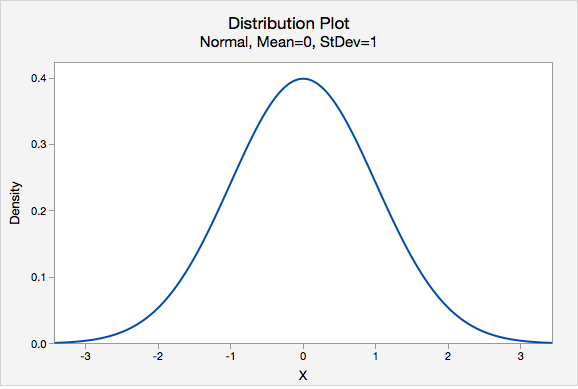# 2.2.7 - The Empirical Rule

A normal distribution is symmetrical and bell-shaped.The Empirical Rule is a statement about normal distributions. Your textbook uses an abbreviated form of this, known as the 95% Rule, because 95% is the most commonly used interval. The 95% Rule states that approximately 95% of observations fall within two standard deviations of the mean on a normal distribution.

Normal Distribution
A specific type of symmetrical distribution, also known as a bell-shaped distribution
Empirical Rule

On a normal distribution about 68% of data will be within one standard deviation of the mean, about 95% will be within two standard deviations of the mean, and about 99.7% will be within three standard deviations of the mean

95% Rule
On a normal distribution approximately 95% of data will fall within two standard deviations of the mean; this is an abbreviated form of the Empirical Rule

## Example: Pulse Rates SectionSuppose the pulse rates of 200 college men are bell-shaped with a mean of 72 and standard deviation of 6.

• About 68% of the men have pulse rates in the interval $$72\pm1(6)=[66, 78]$$.
• About 95% of the men have pulse rates in the interval $$72\pm2(6)=[60, 84]$$.
• About 99.7% of the men have pulse rates in the interval $$72\pm 3(6)=[54, 90]$$.

## Example: IQ Scores Section

IQ scores are normally distributed with a mean of 100 and a standard deviation of 15.

• About 68% of individuals have IQ scores in the interval $$100\pm 1(15)=[85,115]$$.
• About 95% of individuals have IQ scores in the interval $$100\pm 2(15)=[70,130]$$.
• About 99.7% of individuals have IQ scores in the interval $$100\pm 3(15)=[55,145]$$.